• 串联谐振电路品质因数
千次阅读
2017-03-22 10:06:11

在研究各种谐振电路时，常常涉及到电路的品质因素Q值的问题，那末什么是Q值呢？下面我们作详细的论述。

1是一串联谐振电路，它由电容C、电感L和由电容的漏电阻与电感的线电阻R所组成。此电路的复数阻抗Z为三个 元件的复数阻抗之和。

Z=R+jωL+（-j/ωC）=R+j（ωL-1/ωC） ⑴

上式电阻R是复数的实部，感抗与容抗之差是复数的虚部，虚部我们称之为电抗用X表示， ω是外加信号的角频率。

当X=0时，电路处于谐振状态，此时感抗和容抗相互抵消了，即式⑴中的虚部为零，于是电路中的阻抗最小。因此电流最大，电路此时是一个纯电阻性负载电路，电路中的电压与电流同相。电路在谐振时容抗等于感抗，所以电容和电感上两端的电压有效值必然相等，

电容上的电压有效值UC=I*1/ωC=U/ωCR=QU 品质因素Q=1/ωCR，这里I是电路的总电流。

电感上的电压有效值UL=ωLI=ωL*U/R=QU 品质因素Q=ωL/R

因为：UC=UL 所以Q=1/ωCR=ωL/R

电容上的电压与外加信号电压U之比UC/U= （I*1/ωC）/RI=1/ωCR=Q

感上的电压与外加信号电压U之比UL/U= ωLI/RI=ωL/R=Q

从上面分析可见，电路的品质因素越高，电感或电容上的电压比外加电压越高。

电路的选择性：图1电路的总电流I=U/Z=U/［R2+（ωL-1/ωC）2］1/2=U/［R2+（ωLω0/ω0-ω0/ωCω0）2］1/2 ω0是电路谐振时的角频率。当电路谐振时有：ω0L=1/ω0C

所以I=U/{R2+［ω0L（ω/ω0-ω0/ω）］2}1/2= U/{R2+［R2（ω0L/R）2］（ω/ω0-ω0/ω）2}1/2= U/R［1+Q2（ω/ω0-ω0/ω）2］1/2

因为电路谐振时电路的总电流I0=U/R，

所以I=I0/［1+Q2（ω/ω0-ω0/ω）2］1/2有：I/I0=1/［1+Q2（ω/ω0-ω0/ω）2］1/2作此式的函数曲线。设（ω/ω0-ω0/ω）2=Y

曲线如图2所示。这里有三条曲线，对应三个不同的Q值，其中有Q1》Q2》Q3。从图中可看出当外加信号频率ω偏离电路的谐振频率ω0时， I/I0均小于1。Q值越高在一定的频偏下电流下降得越快，其谐振曲线越尖锐。也就是说电路的选择性是由电路的品质因素Q所决定的，Q值越高选择性越好。

更多相关内容
• 在研究各种谐振电路时，常常涉及到电路品质因素Q值的问题，那末什么是Q值呢？本文我们作详细的论述。
• 品质因数谐振电路中一个非常重要的参数, 然而目前国内一般教材对品质因数的定义和计算方 法, 仅限于在简单 RLC 串、并联谐振电路的过压、过流方面
• 谐振电路 品质因数Q 不同定义与推导 能量定义 功率定义 能量定义的功率定义相互转换 系数2π的由来
• 电路模型: 公式： 其中L为电感，单位为亨利（H）；C为电容，单位为法拉（F），R为电阻，单位为欧姆(Ω) 因此将原理图中的参数代入公式，可得： Q = 31.6

电路模型: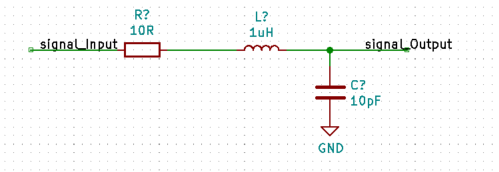公式：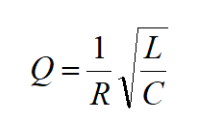其中L为电感，单位为亨利（H）；C为电容，单位为法拉（F），R为电阻，单位为欧姆(Ω)

因此将原理图中的参数代入公式，可得：

Q = 31.6

展开全文嵌入式硬件
• 在研究各种谐振电路时，常常涉及到电路品质因素Q值的问题，那末什么是Q值呢？下面我们作详细的论述。
• ## 电路-品质因数

千次阅读 多人点赞 2021-02-09 17:28:24
品质因数 物理意义 电感线圈、电容器品质因数：用于评价实际电感线圈、电容器...品质因数用来衡量元件质量的好坏，将品质因数扩展到谐振电路，表示谐振电路储能与耗能的比值。 电感线圈的品质因数 线圈电流：i=

## 品质因数

• 物理意义
• 电感线圈、电容器品质因数：用于评价实际电感线圈、电容器的品质。实际电感、电容除储存能量外，都存在一定能量消耗。
• 元件品质因数定义： Q = d e f 2 π 储 能 的 最 大 值 一 周 期 内 的 耗 能 Q \overset{\underset{\mathrm{def}}{}}{=} 2\pi\frac{储能的最大值}{一周期内的耗能}
• 品质因数用来衡量元件质量的好坏，将品质因数扩展到谐振电路，表示谐振电路储能与耗能的比值。

### 电感线圈的品质因数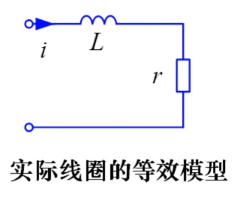• 线圈电流： i = 2 I c o s ω t i = \sqrt{2}Icos\omega t
• 电感储能： w L ( t ) = 1 2 L i 2 = L I 2 c o s 2 ω t w_{L}(t)=\frac{1}{2}Li^{2}=LI^{2}cos^{2}\omega t
• 最大储能为： L I 2 LI^{2}
• 一周期内线圈内阻r的耗能： I 2 r T I^{2}rT
• 电感品质因数： Q L = 2 π L I 2 I 2 r T = 2 π f L r = ω L r Q_{L}=2\pi\frac{LI^{2}}{I^{2}rT}=\frac{2\pi fL}{r} =\frac{\omega L}{r}

### 电容的品质因数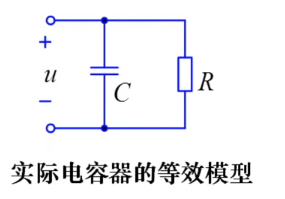• 电容电压： u = 2 U c o s ω t u=\sqrt{2}Ucos\omega t
• 电容储能： w C ( t ) = 1 2 C u 2 = C U 2 c o s 2 ω t w_{C}(t)=\frac{1}{2}Cu^{2}=CU^{2}cos^{2}\omega t
• 最大储能： C U 2 CU^{2}
• 一周期电阻R耗能： U 2 R T \frac{U^{2}}{R}T
• 电容品质因数： Q C = 2 π C U 2 U 2 G T = 2 π f C G = ω C G = ω C R Q_{C}=2\pi\frac{CU^{2}}{U^{2}GT}=\frac{2\pi fC}{G} =\frac{\omega C}{G}=\omega CR

### rLC串联谐振电路中的品质因数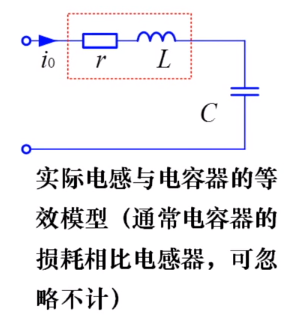• 电路谐振时，回路电流： i = 2 c o s ω 0 t i=\sqrt{2}cos\omega _{0}t
• 电容电压相量： U C 0 ˙ = − j 1 ω 0 C I 0 ˙ = I 0 ω 0 C ∠ 9 0 o \dot{U_{C0}}=-j\frac{1}{\omega _{0}C}\dot{I_{0}}=\frac{I_{0}}{\omega _{0}C}\angle 90^{o}
• 电容电压瞬间式： u c 0 = 2 I 0 ω 0 C c o s ( ω 0 t − 9 0 o ) = 2 I 0 ω 0 C s i n ( ω 0 t ) u_{c0}=\sqrt{2}\frac{I_{0}}{\omega _{0}C}cos(\omega _{0}t-90^{o})=\sqrt{2}\frac{I_{0}}{\omega _{0}C}sin(\omega _{0}t)
• 电感储能： w L 0 ( t ) = 1 2 L i L 0 2 = L I 0 2 c o s 2 ω 0 t w_{L0}(t)=\frac{1}{2}Li_{L0}^{2}=LI_{0}^{2}cos^{2}\omega_{0}t
• 电容储能： w C 0 ( t ) = 1 2 C u C 0 2 = C ( I 0 w 0 C ) 2 s i n 2 ω 0 t w_{C0}(t)=\frac{1}{2}Cu_{C0}^{2}=C(\frac{I_{0}}{w_{0}C})^{2}sin^{2}\omega_{0} t
• 条件：谐振电路中 ω 0 L = 1 ω 0 C \omega_{0}L=\frac{1}{\omega_{0}C}
• 可得电容储能等于电感储能： w C 0 ( t ) = C ( I 0 w 0 C ) 2 s i n 2 ω 0 t = L I 2 s i n 2 ω 0 t w_{C0}(t)=C(\frac{I_{0}}{w_{0}C})^{2}sin^{2}\omega_{0} t=LI^{2}sin^{2}\omega_{0} t
• 谐振电路的总储能为电感储能与电容储能之和： w 0 ( t ) = w L 0 ( t ) + w C 0 ( t ) = L I 0 2 = C U 0 2 , ( 常 数 ) w_{0}(t)=w_{L0}(t) +w_{C0}(t)=LI^{2}_{0}=CU^{2}_{0},(常数)
• 当电感储能最大时电容储能为零，反之亦然。
• 谐振电路谐振时的品质因数： Q = 2 π L I 0 2 I 0 2 r T 0 = w 0 L r = 1 w 0 C r Q=2\pi\frac{LI^{2}_{0}}{I^{2}_{0}rT_{0}}=\frac{w_{0}L}{r}=\frac{1}{w_{0}Cr}
• 谐振电路品质因数反映电路选频特性，品质因数越高，选频特性越好。

### 小结

• 品质因数反映器件和电路的特性。
• 元件串联时，谐振电路品质因数和电阻成反比，电阻越大，品质因数越低。
• 品质因数是衡量谐振电路优势的一个重要参数。
展开全文• ## 电路品质因数Q

万次阅读 2019-05-19 11:33:48
品质因数Q=ULUsQ=\frac{ U_L}{U_s}Q=Us​UL​​,即电感上的电压比上输入电压,是分析和比较谐振电路的一个重要辅助参数。 不同参数的RLC电路在频率响应上的差异，是通过Q值反映出来的。在谐振电路中，它们有以下不同...
• 品质因数 Q = U L U s Q=\frac{ U_L}{U_s} ,即电感上的电压比上输入电压,是分析和比较谐振电路的一个重要辅助参数。
• 不同参数的RLC电路在频率响应上的差异，是通过Q值反映出来的。在谐振电路中，它们有以下不同点
1.它们都在谐振点出现峰值，在其领域都有较大的幅值输出，表明RLC串联电路具有在全频域内选择各自频振信号的性能；
2.当信号频率偏移谐振点，输出信号从峰值下降，表明信号对非谐振频率有抑制作用（简称抑非能力）。抑非能力与Q值成正比，能力越强，通频带越窄，电路选择性越好；能力越弱，通频带越宽，电路选择性变差。

## 二阶传递函数的品质因数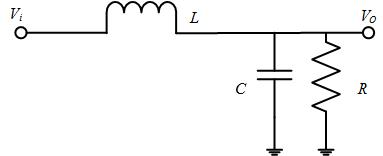• 传递函数 G = V o V i = 1 S 2 L C + S L / R + 1 G={\frac{V_o}{V_i}}={\frac{1}{S^2LC+SL/R+1}}
• 品质因数 Q = R C L Q=R\sqrt{\frac{C}{L}}
LC滤波器伯德图如下图所示：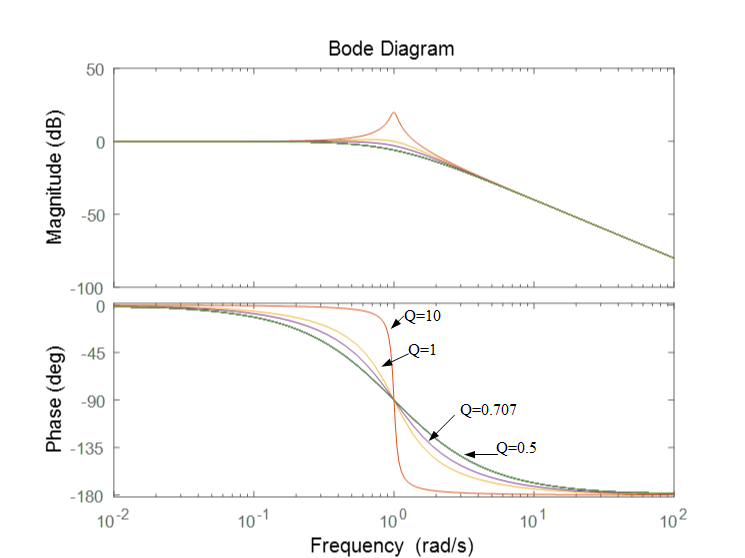• 品质因数Q确定了响应曲线在交接频率处的峰值大小，如图Q=20,那么谐振下输出电压是输入电压的20倍。一般认为Q值越大，则滤波器欠阻尼状态，当Q=0.707，得到临界阻尼，谐振频率的增益比其直流值低3dB。-3dB是0.707的对数，意味着下降约30%。
• 电阻对交接频率影响较小，但其对Q的影响是显著地。L和C的串联寄生电阻越大，Q值越小。
• 工程师一般用阻尼系数 ξ = 1 2 Q ξ={\frac{1}{2Q}} ,高Q值对应小ξ。
展开全文• 总结： （ f0 谐振频率，ω0谐振角频率）  （fc 谐振频率；BW 通频带宽） ...不管是串联还是并联谐振电路，...品质因数谐振电路中的电阻R和电感L或电容C控制，可根据实际应用，通过更改R值来更改谐振电路Q值。
• LC振荡器稳定度与品质因数的关系 影响振荡频率w的参数 Q越大，相同的△φ引起的△ω就越小。 如图所示：Qe’>Qe,△ω’osc1<△ωosc1. 故增加品质因数会增加LC谐振回路的稳定性。
• RLC谐振电路常用简单计算公式 撤销：Ctrl/Command + Z 重做：Ctrl/Command + Y 加粗：Ctrl/Command + B 斜体：Ctrl/Command + I 标题：Ctrl/Command + Shift + H 无序列表：Ctrl/Command + Shift + U 有序列表：Ctrl...单片机 嵌入式硬件
• 品质因数介绍： 在研究各种谐振电路时，常常涉及到电路的品质因素Q值的问题，那末什么是Q值呢？下面我们作详细的论述。 1是一串联谐振电路，它由电容C、电感L和由电容的漏电阻与电感的线电阻R所组成。此电路的...
• 电子政务-带有温度补偿品质因数的集成谐振电路.zip
• 在串联电路中，电路品质因数Q有两种测量方法，一是根据公式 Q=UL/U0=Uc/U0测定，Uc与UL分别为谐振时电容器C与电感线圈L上的电压；另一种方法是通过测量谐振曲线的通频带宽度△f=f2-f1，再根据Q=f0/(f2-f1)求出Q值...
• L C 电路在调谐放大器和L C 振荡电路等很多电子电路中具有十分重要的作用 ,是不可缺少的组成部分，它的性能好坏直接关系到电子设备的质量。为了描述L C 回路的性能,人们引入了一个重要概念即品质因数。但一些教材和...
• 电路品质因数应为存储的能量与一个周期内消耗的能量的比值的 2 π 2\pi 2π倍 以 R L C RLC RLC并联谐振电路为为例 电路总导纳： Y = 1 R + 1 j ω L + j ω C {Y}=\frac{1}{R}+\frac{1}{j\omega L}+j\omega C Y=...
• 摘 要： 从RLC 串联谐振电路的方程分析出发， 推导了电路谐振状态下的谐振频率、通频带、品质因数和输入阻抗， 并且基于Multisim 10 仿真软件创建RLC 串联谐振电路， 利用其虚拟仪表和仿真分析， 分别用测量及仿真...
• Q表的工作原理是在串联谐振电路上，每个电抗元件两端的电压是作用于谐振回路电压的Q倍。故Q值可以直接由两个电压的比值来决定。 　测试电路如图（a）所示。在测量过程中，一定要注意施加的电压不要使电感两端的电压...
• 在研究各种谐振电路时，常常涉及到电路品质因素Q值的问题，那末什么是Q值呢？下面我们作详细的论述。 1是一串联谐振电路，它由电容C、电感L和由电容的漏电阻与电感的线电阻R所组成。此电路的复数阻抗Z为三个元件...
• 在学习通信电子线路的过程中，起初只是把品质因数（品质因子Q）理解成一个人为规定好的无量纲参数，并未再多关注。 实际上，品质因子是物理、工程上衡量振子阻尼性质的物理量和振子的共振频率相对于带宽的大小的重要...
• 摘要：基于探索RLC串联电路谐振特性仿真实验技术的目的，采用Multisim10仿真软件对RLC串联电路谐振特性进行了仿真实验测试，给出了几种Multisim仿真实验方案，介绍了谐振频率、上限频率、下限频率及品质因数的测试和...
• ## 一阶LC谐振电路

千次阅读 2021-01-29 08:20:10
一阶LC谐振电路 　LC谐振电路具有选频功能，广泛用于各种通信电路。本文就一阶LC电路进行仿真，以此来进一步加深对电路特性的理解和记忆。 谐振 　当电路中存在感性元件（如电感）或者容性元件（电容）时，信号源...
• 基于探索 RLC串联电路谐振特性仿真实验技术的目的，采用Multisim10仿真软件对RLC串联电路谐振特性进行了仿真实验测试，给出了几种Multisim仿真实验方案，介绍了谐振频率、上限频率、下限频率及品质因数的测试和计算...
• CLL谐振变换器谐振网络电路参数的设计关系到变换器性能的好坏。基于基波近似法，并结合特征阻抗分析方法，提出一种CLL谐振变换器谐振网络电路参数优化设计方案。详细分析了CLL谐振变换器的直流增益特性、谐振网络...
• 在许多方面，并联谐振电路正是如此与我们在前一个教程中看到的串联谐振电路相同。两者都是3元件网络，包含两个无功元件，使它们成为二阶电路，两者都受到电源频率变化的影响，两者都有一个频率点，它们的两个电抗...
• 摘 要： 从RLC 串联谐振电路的方程分析出发， 推导了电路谐振状态下的谐振频率、通频带、品质因数和输入阻抗， 并且基于Multisim 10 仿真软件创建RLC 串联谐振电路， 利用其虚拟仪表和仿真分析， 分别用测量及仿真......